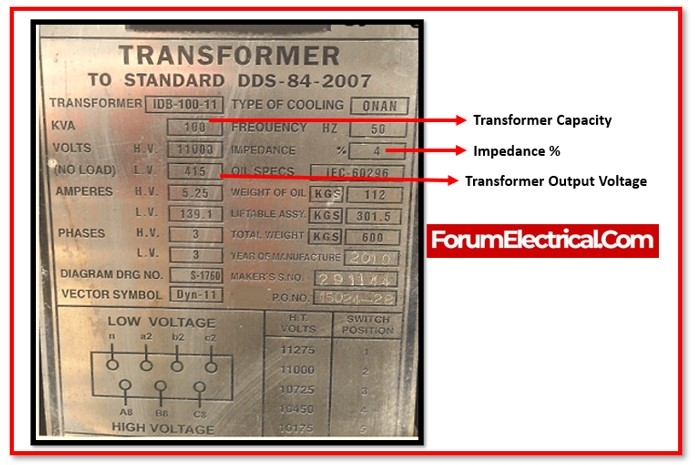# Optimizing Power Systems for Large Motor Startup: Transformer Sizing and Voltage Drop Analysis

0
217Determining the size of a transformer & the voltage drop caused by starting a large-sized motor includes various factors and necessitates specific details about the motor & the system.

The following is a general summary of all the stages involved

# Transformer Sizing Considerations

The transformer‘s size should allow it to start a motor with a higher current without experiencing an excessive voltage drop. It is typical practice to size the transformer to withstand a voltage drop of no more than 5 to 10 percent while starting a motor.

Following are the steps to calculate the transformer rating:

Full Load Current of a Transformer = KVA/(√3 X Secondary Voltage)

ILX=KVA/(1.732xV2)

Short Circuit Current of a Transformer = Transformer Full Load Current / Impedance

Isc=ILX/z

Analysing Motor Parameters

• Identify the motor’s rated power (in kW or HP).
• Find the rated voltage (in V) for the motor.
• Find the motor’s power factor (typically listed on the nameplate or datasheet of the motor).
• Take note of the starting method used by the motor (Star-Delta, Direct-on-Line,  Soft Starter, VFD, etc.).

Motor Full Load Current = (Motor Size x 1000)/( √3 x Motor Voltage x Power Factor)

ILM=(KW x 1000)/(1.732 x VM x P.F)

# Voltage Drop of a Transformer (in Secondary)

The following formula can be used to estimate the voltage drop of a transformer produced by the inrush current of a motor & the maximum KVA rating of the transformer:

VD=IRSM/Q1

Where,

VD = Voltage Drop

IRSM = Inrush Current

Q1= Maximum KVA of Transformer at Short Circuit

# Motor Code

An electric motor’s type and features are identified using motor codes. They are often made up of a series of letters & numbers, each representing a distinct aspect of information.

Motor codes are a useful tool in identifying & selecting the appropriate electric motor for a given application.